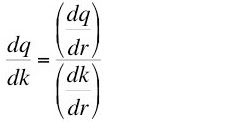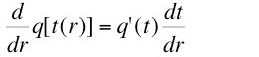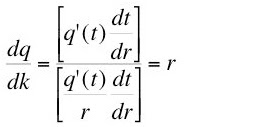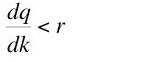## Monday, March 12, 2007

### Interest Rate Still Unequal to Marginal Product of Capital (Part 2/2)

4.0 Wicksell Effects
The model from the previous post shows how the value of output and of capital per worker depend on the interest rate. In effect, I have traced out a parametric specification of a continuously differentiable aggregate neoclassical production function q(k), where both output and capital per capita are specified in common units. This production function can be differentiated:(13)
The numerator is easily found:(14)

The denominator is more interesting. From the chain rule for differentiation, one obtains:(15)
The first term on the right shows the variation in the value of capital by a change in the optimal technique. This variation is known as the real Wicksell effect. The optimal technique changes with the interest rate, although the real Wicksell effect is evaluated at a given value of the interest rate. The second term on the right hand side of Equation 15 shows the variation of the value of capital with changes in the interest rate, given the technique. This term is the price Wicksell effect.

Wicksell effects can be calculated for this particular model. The price Wicksell effect is easily found from Equation 7:(16)
For this simple model, the price Wicksell is always positive:(17)
The determination of the real Wicksell effect requires the calculation of the partial derivative of the value of capital with respect to its first argument, the optimal process length:(18)
Evaluating for the optimal process length, as given by Equation 10, this partial derivative is considerably simplified:(19)

Suppose price Wicksell effects were zero, which they are not. Then one would have for the marginal product of capital in this model:(20)
But, since price Wicksell effects are positive, the denominator in Equation 13 exceeds the real Wicksell effect. Consequently, the marginal product of capital is, in equilibrium, less than the interest rate in this model:(21)
Because of non-zero price Wicksell effects, the marginal product of capital is generally unequal to the interest rate.

One generally cannot place any restriction on the direction of (price or real) Wicksell effects. They may be either positive or negative. Austrian-Wicksell flow-input flow-output models and the Sraffian analysis of the choice of technique are two examples of classes of models I consider reasonably general.

Suppose one were only willing to consider model solutions in which the real Wicksell effect is negative. That is, one considers variations in the optimal technique with changes in the interest rate. One assumes that the value of the newly selected capital goods, evaluated at non-varying prices, is such that a higher interest rate is associated with a lower capital intensity. If one does make Burmeister's arbitrary regularity assumption, Chmpernowne's chain index for capital is well-defined. This chain index is found by adding up real Wicksell effects from an interest rate of zero to the interest rate in which one is interested. Using this measure and a corresponding chain index for output, the equilibrium interest rate is equal to the marginal product of capital. One should note that the wage is no longer equal to (discounted) marginal product of labor for these measures. Nor are they empirically observable. I don't think that this approach is all that interesting; its only purpose seems to be to defend the failed aggregate neoclassical model.

Notice that the above analysis uses a smooth aggregate production function. In the model, the value of all capital goods is easily aggregated in the same units of measurement as the single consumption good. The value of capital is a well-defined quantity. All firms are identical, and net output consists of a single commodity. The modeling of production makes it apparent that the inequality between the marginal product of capital and the interest rate is not driven in other models by adopting a discrete model of production. The inequality is the result of non-zero price Wicksell effects.

References
• Ahmad, Syed (1991). Capital in Economic Theory: Neo-Classical, Cambridge, and Chaos, Edward Elgar
• Baldone, Slavatore (1984). "From Surrogate to Pseudo Production Functions," Cambridge Journal of Economics, V. 8: 271-288
• Bidard, Christian (2000). "Wicksell and Douglas on Distribution and Marginal Productivity," in Critical Essays on Piero Sraffa's Legacy in Economics (edited by H. D. Kurz), Cambridge University Press
• Burmeister, Edwin (1987). "Wicksell Effects", in The New Palgrave (edited by J. Eatwell, M. Milgate, and P. Newman), Macmillan